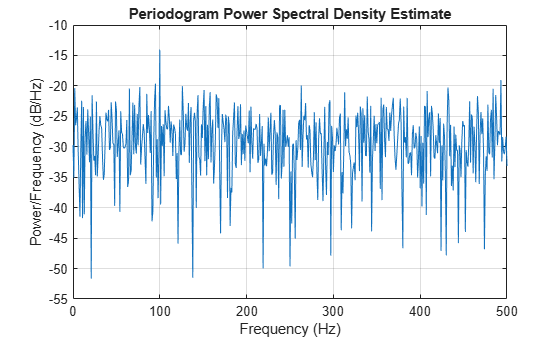Documentation

## Significance Testing for Periodic Component

This example shows how to assess the significance of a sinusoidal component in white noise using Fisher's g-statistic. Fisher's g-statistic is the ratio of the largest periodogram value to the sum of all the periodogram values over 1/2 of the frequency interval, (0, `Fs`/2). A detailed description of the g-statistic and exact distribution can be found in the references.

Create a signal consisting of a 100 Hz sine wave in white Gaussian noise with zero mean and variance 1. The amplitude of the sine wave is 0.25. The sample rate is 1 kHz. Set the random number generator to the default settings for reproducible results.

```rng default Fs = 1e3; t = 0:1/Fs:1-1/Fs; x = 0.25*cos(2*pi*100*t)+randn(size(t));```

Obtain the periodogram of the signal using `periodogram`. Exclude 0 and the Nyquist frequency (`Fs`/2). Plot the periodogram.

```[Pxx,F] = periodogram(x,rectwin(length(x)),length(x),Fs); Pxx = Pxx(2:length(x)/2); periodogram(x,rectwin(length(x)),length(x),Fs)```Find the maximum value of the periodogram. Fisher's g-statistic is the ratio of the maximum periodogram value to the sum of all periodogram values.

```[maxval,index] = max(Pxx); fisher_g = Pxx(index)/sum(Pxx)```
```fisher_g = 0.0381 ```

The maximum periodogram value occurs at 100 Hz, which you can verify by finding the frequency corresponding to the index of the maximum periodogram value.

```F = F(2:end-1); F(index)```
```ans = 100 ```

Use the distributional results detailed in the references to determine the significance level, `pval`, of Fisher's g-statistic. The following MATLAB® code implements equation (6) of . Use the logarithm of the gamma function to avoid overflows when computing binomial coefficients.

```N = length(Pxx); nn = 1:floor(1/fisher_g); I = (-1).^(nn-1).*exp(gammaln(N+1)-gammaln(nn+1)-gammaln(N-nn+1)).*(1-nn*fisher_g).^(N-1); pval = sum(I)```
```pval = 2.0163e-06 ```

The p-value is less than 0.00001, which indicates a significant periodic component at 100 Hz. The interpretation of Fisher's g-statistic is complicated by the presence of other periodicities. See  for a modification when multiple periodicities may be present.

References

 Percival, Donald B. and Andrew T. Walden. Spectral Analysis for Physical Applications. Cambridge, UK: Cambridge University Press, 1993.

 Wichert, Sofia, Konstantinos Fokianos, and Korbinian Strimmer. "Identifying Periodically Expressed Transcripts in Microarray Time Series Data." Bioinformatics. Vol. 20, 2004, pp. 5-20.